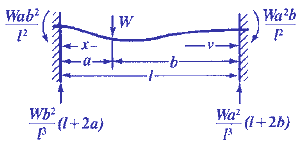Bending, Deflection and Stress Equations Calculator for
Fixed at Both Ends, Load at any location

Beam Deflection and Stress Formula and Calculators

Structural Beam Deflection, Stress, Bending Equations and calculator for a Beam Fixed at Both Ends, Load at any location.Moment and Loads at Supports

M = Wab2 / l2
W1=(Wb2 / l3))(l + 2a)

Section Modulus Z

Z = I/z

I = Moment of inertia Cross Section : Units in4 (m4)
z = Distance neutral axis to extreme fiber: Units in (m)

General Stress at any Given Point

For segment length a,For segment length bStress at Critical Locations/Points

Stress at end nearest to segment of length a,Stress at end nearest to segment of length b,Maximum stress is at end nearest to shorter segment.
Stress is zero atandGreatest negative stress, at loadDeflection at any Point

For length a,For length bCritical DeflectionsIf b length is the longer segment and a is the shorter one.

The maximum deflection is in the longer segment:andWhere:

 E = Modulus of Elasticity psi (N/mm2) I = Moment of Inertia in4 (mm4) W = Load lbs (N) s = Stress at the cross-section being evaluated Lbs/in2 (N/mm2) y = Deflection inches (mm) x = Some distance as indicated inches (mm) u = Some distance as indicated inches (mm) a, b = Some distance as indicated inches (mm) L = Some distance as indicated inches (mm)= Some distance as indicated inches (mm) Z = section modulus of the cross-section of the beam = I/z in3 (mm3) z = distance from neutral axis to extreme fiber (edge) inches (mm)
• Please note letter "" (lower case "L") is different than "I" (Moment of Inertia).
• Deflections apply only to constant cross sections along entire length.

References:

• Any Machinery's Handbook published since 1931 or,
• Machinery's Handbook, 21st Edition
• Machinery's Handbook, 23st Edition
• Machinery's Handbook, 27st Edition© Copyright 2000 - 2019, by Engineers Edge, LLC www.engineersedge.comMembership Register | LoginHomeEngineering Book StoreEngineering ForumExcel App. DownloadsOnline Books & ManualsEngineering NewsEngineering VideosEngineering CalculatorsEngineering ToolboxGD&T Training Geometric Dimensioning TolerancingDFM DFA TrainingTraining Online EngineeringAdvertising CenterCopyright Notice